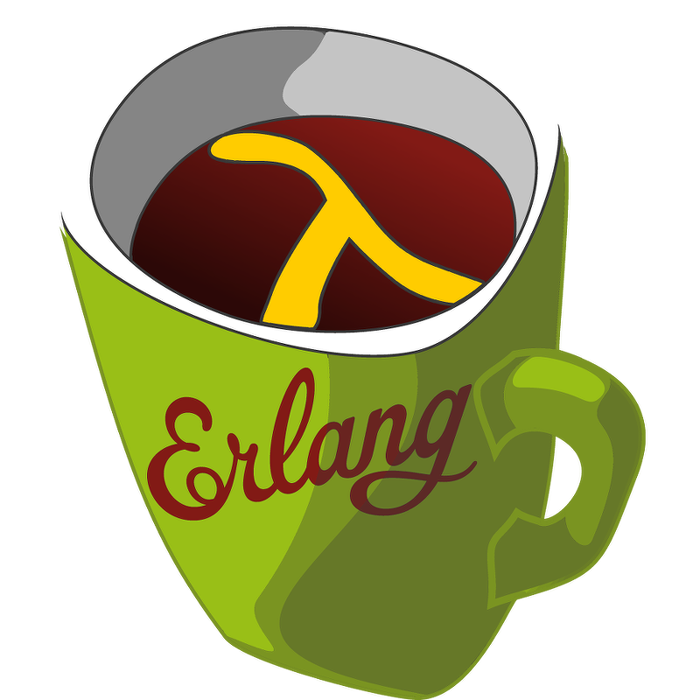This week's LFE Friday was translated with permission from the Erlang Thursday series by Steven Proctor. This week's translator: Robert Virding.

Today’s LFE Friday is lists:dropwhile/2.

`lists:dropwhile/2` takes a predicate function and a list, and returns a list where the first series of items for which the predicate function returned `true` have been removed.

``````> (lists:dropwhile #'erlang:is_atom/1 '(hello World 1 3 4))
(1 3 4)
> (lists:dropwhile (lambda (x) (> x 0)) '(-1 0 1 2 3))
(-1 0 1 2 3)
> (lists:dropwhile (lambda (x) (> x 0)) '(-2 -1 0 1 2 3))
(-2 -1 0 1 2 3)
> (lists:dropwhile (lambda (x) (< x 0)) '(-2 -1 0 1 2 3))
(0 1 2 3)
> (lists:dropwhile (lambda (x) (< x 0)) '(0 -1 -2 -3 -4 -5))
(0 -1 -2 -3 -4 -5)
> (lists:dropwhile (lambda (x) 'true) '(hello World 1 3 bar 4))
()
> (lists:dropwhile (lambda (x) 'false) '(hello World 1 3 bar 4))
(hello World 1 3 bar 4)``````

Unlike lists:filter/2, `lists:dropwhile/2` stops checking the list as soon as the predicate function returns `false`. This means that elements for which the predicate function would return `true` can still appear in the result list, as if they occur after an element for which the predicate function returns `false`.

``````> (lists:dropwhile #'erlang:is_atom/1 '(hello World foo 1 3 bar 4))
(1 3 bar 4)
> (lists:filter (lambda (x) (not (is_atom x))) '(hello World foo 1 3 bar 4))
(1 3 4)
> (lists:dropwhile (lambda (x) (< x 0)) '(-2 -1 0 1 -5 3 7))
(0 1 -5 3 7)
> (lists:filter (lambda (x) (>= x 0)) '(-2 -1 0 1 -5 3 7))
(0 1 3 7)``````

–Proctor, Robert

10 February 2015

tutorials